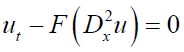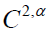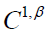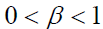#### 期刊菜单

A Priori Estimates for Classical Solutions of Fully Non-linear Parabolic Equations
DOI: 10.12677/PM.2012.24038, PDF, HTML, 下载: 3,208  浏览: 10,296  国家自然科学基金支持

Abstract: For the fully nonlinear uniformly parabolic equations.It is well known that the viscosity solutions are ofif the nonlinear operators are convex (or concave). In this paper, we study the classical solution for the fully nonlinear parabolic equations, where the nonlinear operators F is localalmost everywhere for.It will be shown the interiorregularity of the classical solutions provided there exists a function ρ that is a continuous modulus of second order derivatives of the classical solution.

  L. H. Wang. On the regularity of fully nonlinear parabolic equations: I. Communications on Pure and Applied Mathematics, 1992, 45: 27-76.  L.C. Evans. Classical solutions of fully nonlinear, convex, second-order elliptic equations. Communications on Pure and Applied Mathematics, 1982, 35(3): 333-363.  L. A. Caffarelli, X. Cabre. Fully nonlinear elliptic equations. American Mathematical Society, 1995.  曹毅, 王立周. 完全非线性椭圆方程的古典解[J]. 数学年刊, 2010, 31: 557-564.  G. M. Lieberman. Second order parabolic partial differential equation. Iowa State: Would Scientific, 1996.  陈亚浙. 二阶抛物型偏微分方程[M]. 北京: 北京大学出版社, 2003.